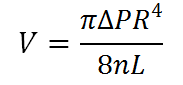# Volumetric Flow Rate using Poiseuilles Equation Calculator

This CalcTown calculator calculates the Volumetric Flow Rate of a liquid using Poiseuilles Equation.

Pascal
m
Pa.s
m

#### Result

Meters/Secondwhere

R: Cross-sectional radius of the pipe
ΔP: Pressure difference of two ends, in Pascal
η: Viscosity of the fluid, in Pa.s
L: Pipe length, in meter
V: Volumetric Flow Rate, in m/s